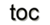Next: 1.2 Physical Fields and Up: 1. Mathematical Concepts Previous: 1. Mathematical Concepts

# 1.1 Introduction

One of the most fundamental concepts in scientific computing is the numerical and discrete treatment of the transformation of physical field problems into mathematical problems and then into the finite and discrete area of computational feasible algorithms and data structures. The reason for the following sections and definitions is therefore to extract the relevant concepts for scientific computing based on mathematical concepts to provide:

• a proven foundation
• a roadmap for application design
• great flexibility and longevity of resulting software

The foundation and roadmap for application design are initiated by the separation of two important concepts based on the topic of algebraic topology. First, space or, in a more general way, space-time has to be discretized into simple computable blocks, e.g., into a collection of cells describable by the theory of cell complexes and their topological structure. An algebraic description is then presented by the concept of chains. Second, concepts from (co)homology are introduced to handle the different types of physical quantities with their respective dimension and orientation by algebraic structures and thus make computer resources available for simulation. Selected concepts from topology and fiber bundles are then applied to specify the transition between continuous space and the concept of differential forms and the finite regime of computer simulation in a formal way. Topology, chains, and the fiber model, which are introduced in this chapter, are axiomatic studies. This results in the fact that a large number of definitions is required.

The contribution of this section is the preparation of basic formal mechanisms for the methodology of discretization schemes introduced subsequently. Structural information and guidelines for applied numerical analysis can thus be obtained. The result of this chapter is a foundation for a formal language used in the following chapters, which builds a framework to introduce the common concepts hidden under the surface of a variety of physical and mathematical modeling concepts, commonly represented by equations of different types, thereby enabling new possibilities for specification and optimization of developed algorithms.Next: 1.2 Physical Fields and Up: 1. Mathematical Concepts Previous: 1. Mathematical Concepts

R. Heinzl: Concepts for Scientific Computing